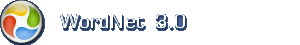English dictionary

Hint: With the Firefox addon you can search this dictionary from the browsers search field.

English noun: single-valued function

1. single-valued function (mathematics) a mathematical relation such that each element of a given set (the domain of the function) is associated with an element of another set (the range of the function)

Synonymsfunction, map, mapping, mathematical function

Narrower (hyponym)circular function, expansion, exponential, exponential function, inverse function, isometry, Kronecker delta, metric, metric function, multinomial, operator, polynomial, threshold function, transformation, trigonometric function

Domain categorymath, mathematics, maths